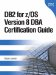# CASE Expressions

### CASE Expressions

You can add if-then-else logic to your SQL statements and output by using CASE expressions. Consider the generation of a list of those candidates who have passed the DB2 Fundamentals exam. In the report, you want to print the scores of the tests, but instead of printing the numeric scores, you want to print a message. If the score is below 65, you want to print Not Passed. If it is between 65 and 90, you want to print Passed, and if the score is above 90, you want to print Excellent. The following SQL statement using a CASE expression accomplishes this:

` SELECT FNAME,LNAME,    CASE       WHEN SCORE < 65 THEN 'NOT PASSED'       WHEN SCORE <= 90 THEN 'PASSED '       ELSE       'EXCELLENT'    END AS TEST_RESULT FROM CANDIDATE C, TEST_TAKEN TT WHERE C.CID=TT.CID AND TT.NUMBER='500'; `

This SQL statement provides string messages based on the conditions of the CASE expression. In this example, the score column features a numeric value, but we use it to produce a character string. The column derived from the CASE expression has been assigned the name TEST_RESULT.

The order of the conditions for the CASE expression is very important. DB2 will process the first condition first, then the second, and so on. If you do not pay attention to the order in which the conditions are processed, you might be retrieving the same result for every row in your table. For example, if you coded the <= 90 option before the < 65, all the data that is lower than 91, even 64 or 30, would display the message Passed.

CASE expressions can be used in places other than select lists, such as WHERE, ON, and HAVING clauses. CASE expressions can also be nested within other CASE expressions.

NOTE

You must use the END keyword to finish a CASE statement.

#### Using CASE Expressions in Functions

CASE expressions can be embedded as function parameters, allowing you to pass various parameters to the function in a single pass of the data. Suppose that the TEST_TAKEN table is very large and that the following need to be counted:

• The number of tests taken with a score higher than 90

• The number of tests taken with a score of 90

• The number of tests taken with a score lower than 70

• The number of DB2 Fundamentals exams taken

Without the use of CASE expressions, the count will require four queries that will potentially read the entire table. We want to do this in a single pass of the data, because the table is very large. To simulate the COUNT function, the query will use four SUM functions, each one evaluating different criteria, using a CASE expression:

` SELECT SUM (CASE WHEN SCORE > 90 THEN 1    ELSE NULL    END) AS MOREGB90,    SUM (CASE WHEN SCORE = 90 THEN 1    ELSE NULL    END) AS EQUALGB90,    SUM (CASE WHEN SCORE < 70 THEN 1    ELSE NULL    END) AS MINORGB70,    SUM (CASE WHEN NUMBER='500' THEN 1    ELSE NULL    END) AS EQUALGB500 FROM TEST_TAKEN WHERE SCORE >= 90    OR SCORE < 70    OR NUMBER = '500'; `

This type of query may be useful for performing data inspection analysis. Note that the four requirements are solved in a single pass of the data. The query was created using a different SUM function for each one of the conditions presented as a requirement. The conditions are evaluated in the CASE expression inside each function. When the condition evaluates trUE, it will return a value of 1, and the row will be summed. When the condition evaluates FALSE, the CASE expression will return a null value, and the row will not be summed.

NOTE

It is important to remember to code the WHERE clause predicate. Although rows that do not qualify will not affect the result of the SUM functions, DB2 will still apply the CASE expression to these rows. If these rows are excluded by the WHERE clause, performance will be improved by avoiding unnecessary CASE expressions against these rows.DB2 for z/OS Version 8 DBA Certification Guide
ISBN: 0131491202
EAN: 2147483647
Year: 2003
Pages: 175
Authors: Susan Lawson